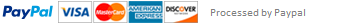Python Homework 4

# Put your name in this modeul-level variable

# Create a class that has two attributes:

# a "name" variable that contains a (str)

# a "scores" variable that will be a list of numbers

class Student:

# I should be able to instantiate a class by saying

# student = quiz4.Student('Mark', [94, 98, 23])

# I should then be able to access

# student.name

# student.scores

#

# Hint: recall the __init__ special functions

pass

# I should be able to get a student's average score

# by avg = student.average()

# This means you need a class instance method here

def average(___):

pass

# I should be able to get a tuple:

# high, low, count = student.summary()

# High would be the high score, low the lowest, and count the number

# of scores belonging to a student.

# A student with no scores should return (None, None, 0)

# This means you need a class instance method here

def summary(___):

pass

# I should be able to add a score to a student's list of scores

pass

# For the methods above: remember the "self" argument!

# Write some test cases! After adding a score, the values

# that the other functions produce should change when called

# Create a module-level function that returns the sum of squares

# of a given list of numbers

def sum_of_squares(___):

pass

This assignment has been answered 3 times in private sessions.